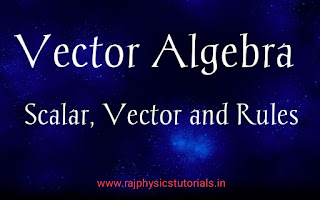## Vector Algebra || Vector Algebra Laws || CSIR NET/JRF, IIT JAM, GATE, JEST,TIFR,SET,DU,BHU,JNU Physics Entrance Exams Study materials

We know that scalar and vector quantities are very important for every physics and maths exams.

## Scalar

A quantity having only magnitude is called Scalar. eg.- distance, speed etc

## Vector

A quantity having both magnitude and direction. A vector is represented by an arrow. The length of the arrow gives the magnitude of the vector and the direction of the vector.

Notation- it is generally represented by A vector or A.

Example- Displacement, Velocity, Force etc.Vector Algebra